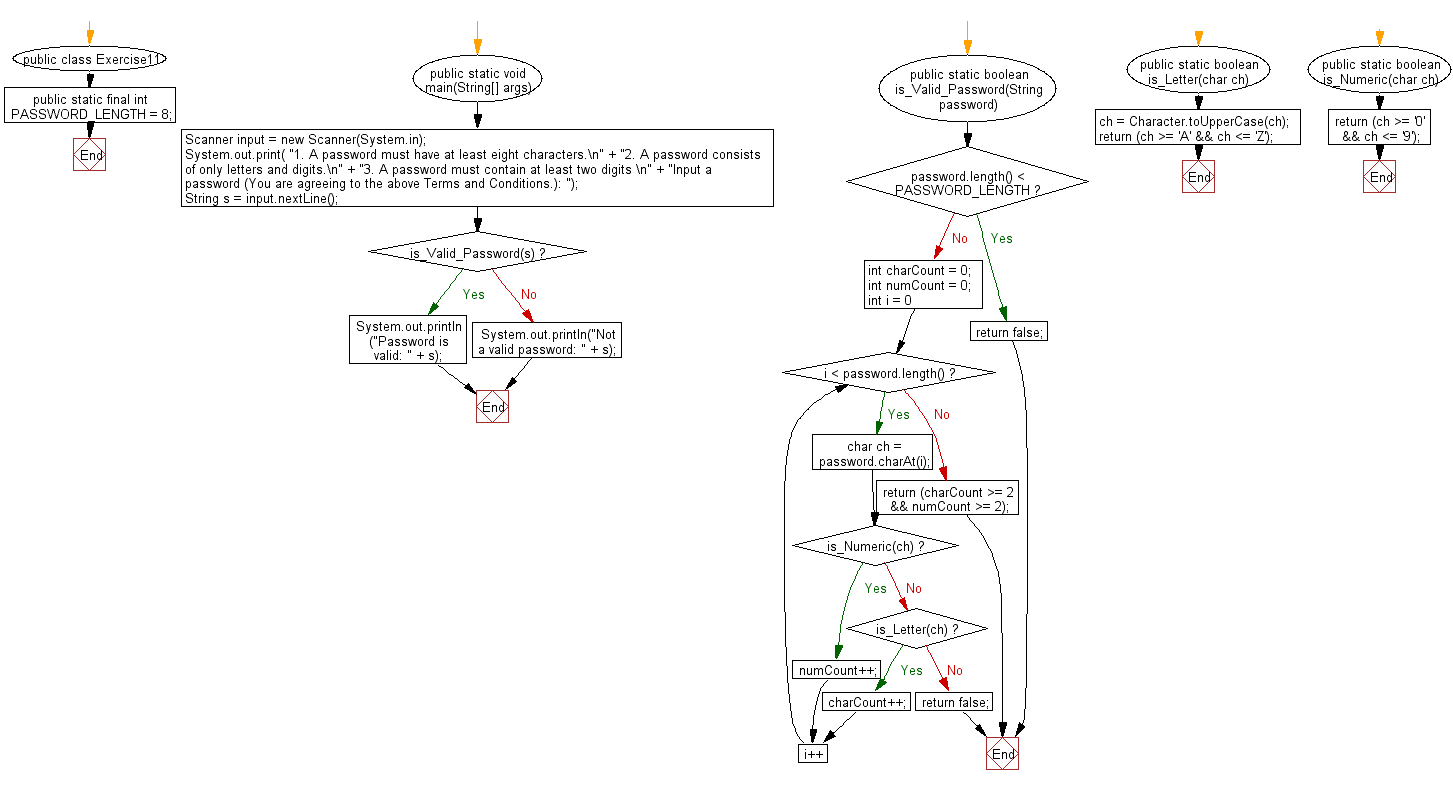﻿ Java exercises: Check whether a string is a valid password - w3resource# Java Method Exercises: Check whether a string is a valid password

## Java Method: Exercise-11 with Solution

Write a Java method to check whether a string is a valid password.

A password must have at least ten characters.
A password consists of only letters and digits.
A password must contain at least two digits.

Sample Solution:

Java Code:

``````import java.util.Scanner;

public class Exercise11 {

public static final int PASSWORD_LENGTH = 8;

public static void main(String[] args) {

Scanner input = new Scanner(System.in);
System.out.print(
"1. A password must have at least eight characters.\n" +
"2. A password consists of only letters and digits.\n" +
"3. A password must contain at least two digits \n" +
"Input a password (You are agreeing to the above Terms and Conditions.): ");
String s = input.nextLine();

System.out.println("Password is valid: " + s);
} else {
System.out.println("Not a valid password: " + s);
}

}

int charCount = 0;
int numCount = 0;
for (int i = 0; i < password.length(); i++) {

if (is_Numeric(ch)) numCount++;
else if (is_Letter(ch)) charCount++;
else return false;
}

return (charCount >= 2 && numCount >= 2);
}

public static boolean is_Letter(char ch) {
ch = Character.toUpperCase(ch);
return (ch >= 'A' && ch <= 'Z');
}

public static boolean is_Numeric(char ch) {

return (ch >= '0' && ch <= '9');
}

}
```
```

Sample Output:

```1. A password must have at least eight characters.
2. A password consists of only letters and digits.
3. A password must contain at least two digits
Input a password (You are agreeing to the above Terms and Conditions.): abcd1234